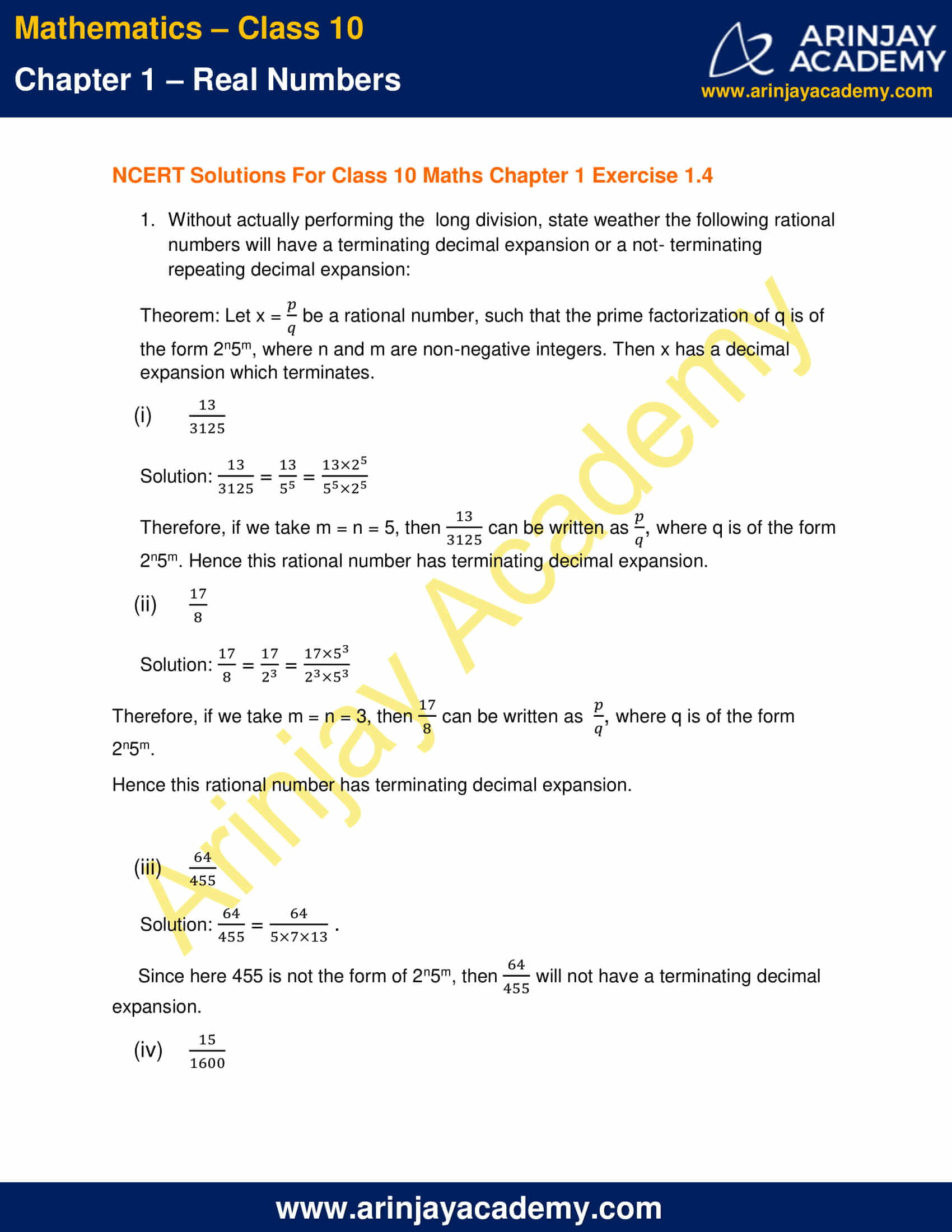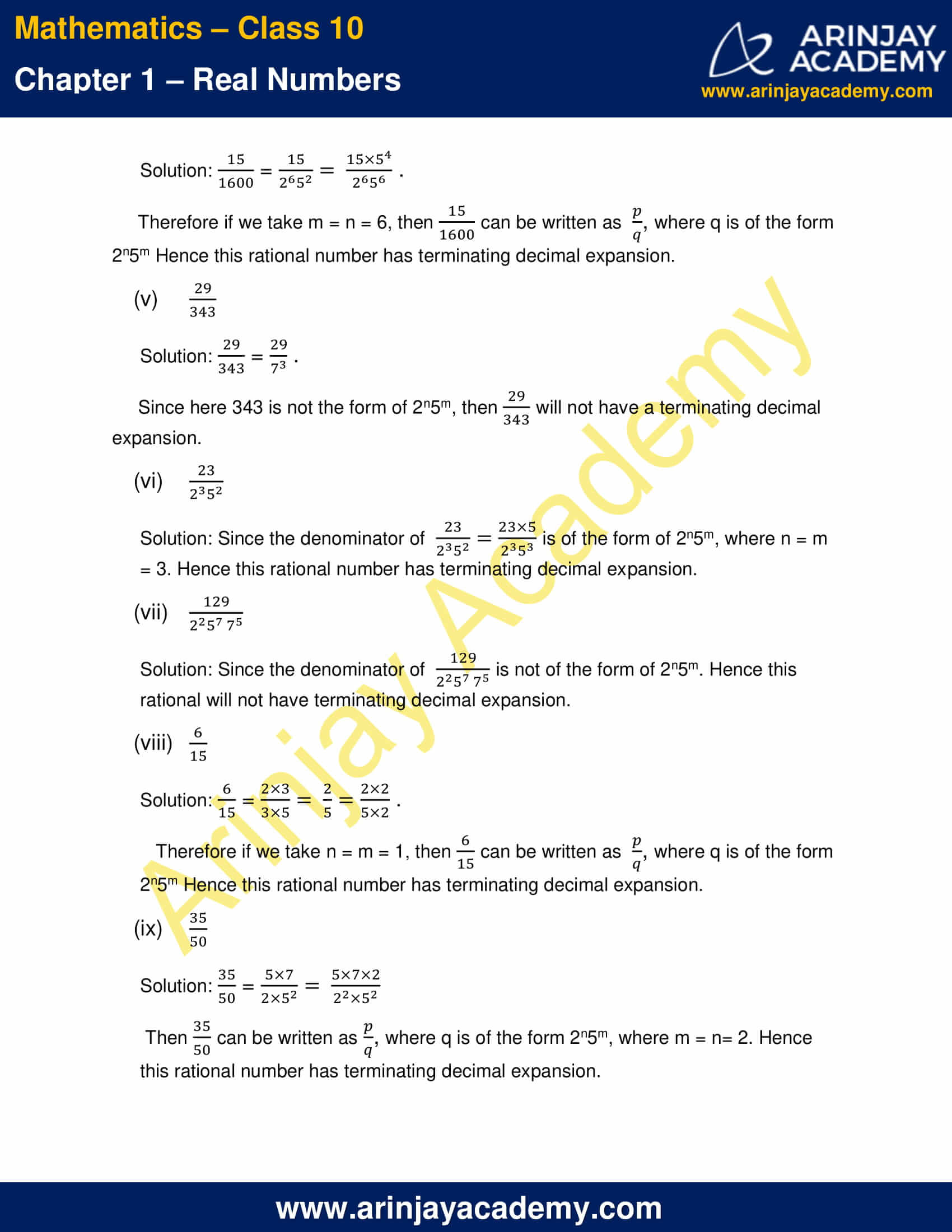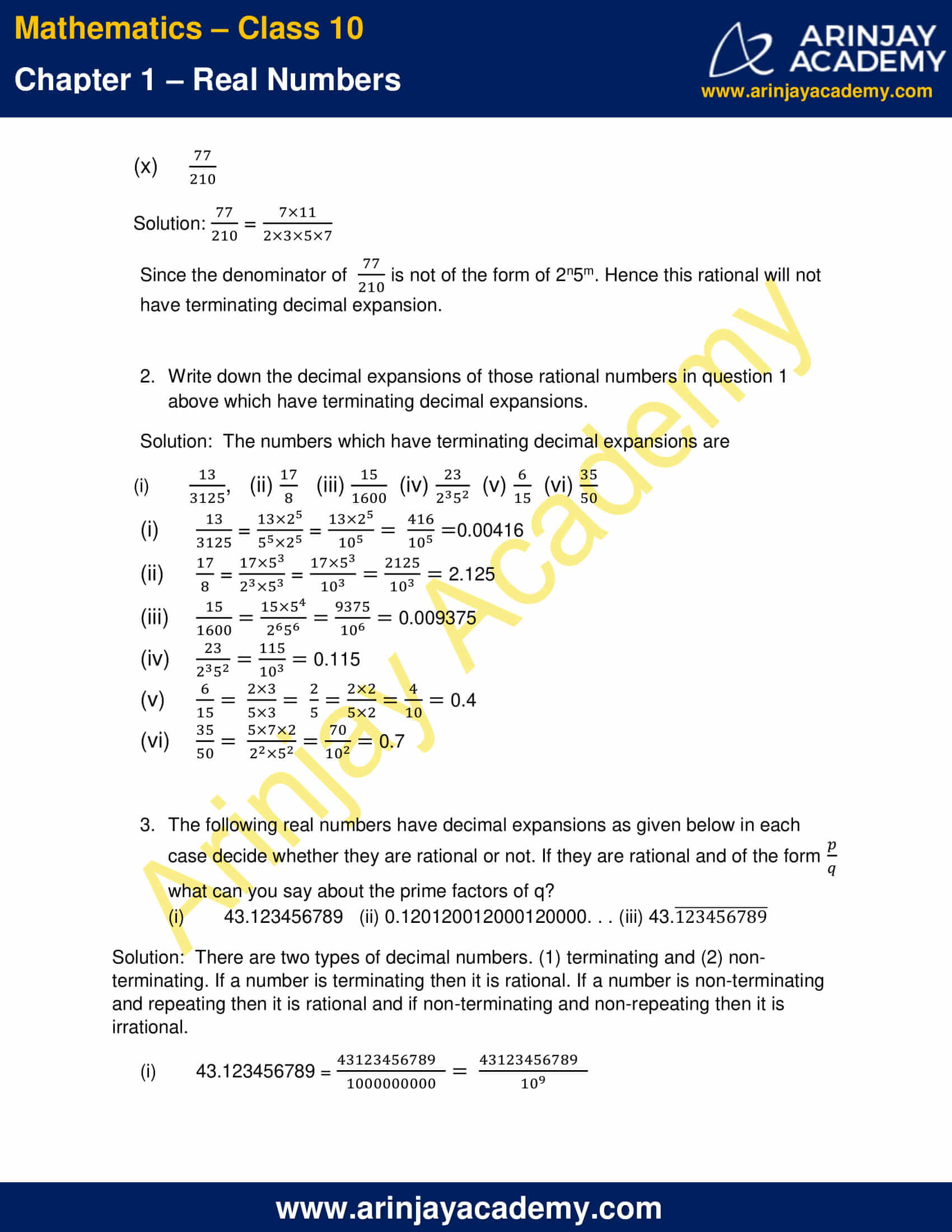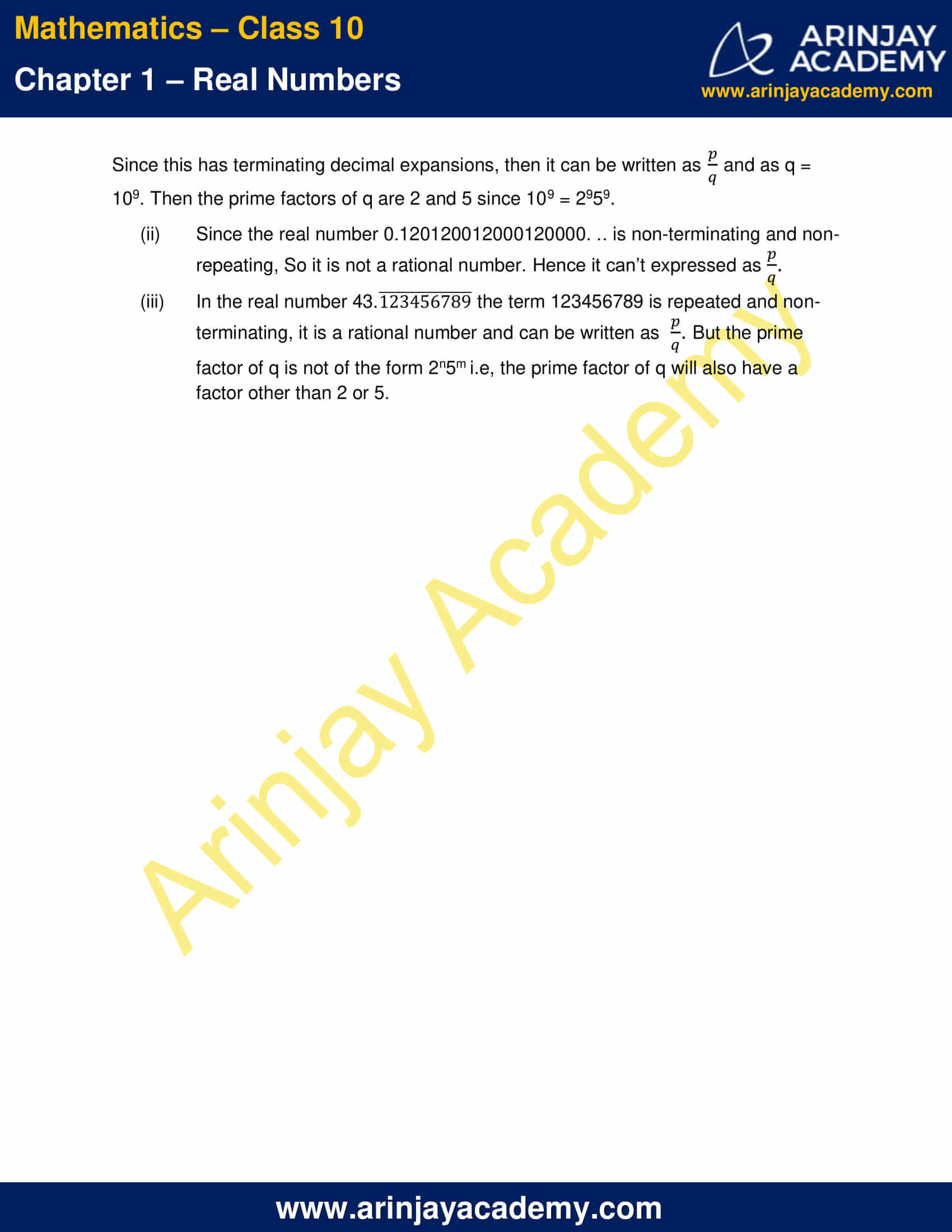# NCERT Solutions For Class 10 Maths Chapter 1 Exercise 1.4 – Real Numbers

Download NCERT Solutions For Class 10 Maths Chapter 1 Exercise 1.4 – Real Numbers. This Exercise contains 3 questions, for which detailed answers have been provided in this note. In case you are looking at studying the remaining Exercise for Class 10 for Maths NCERT solutions for other Chapters, you can click the link at the end of this Note.### NCERT Solutions For Class 10 Maths Chapter 1 Exercise 1.4 – Real NumbersNCERT Solutions For Class 10 Maths Chapter 1 Exercise 1.4 – Real Numbers

1. Without actually performing the long division, state weather the following rational numbers will have a terminating decimal expansion or a not- terminating repeating decimal expansion:

Theorem: Let x =$\cfrac { p }{ q }$ be a rational number, such that the prime factorization of q is of the form 2n5m, where n and m are non-negative integers. Then x has a decimal expansion which terminates.

(i)$\cfrac { 13 }{ 3125 }$

Solution:$\cfrac { 13 }{ 3125 }$ =$\cfrac { 13 }{ { 5 }^{ 5 } }$ =$\cfrac { 13\times { 2 }^{ 5 } }{ { 5 }^{ 5 }\times { 2 }^{ 5 } }$

Therefore, if we take m = n = 5, then$\cfrac { 13 }{ 3125 }$ can be written as$\cfrac { p }{ q }$, where q is of the form 2n5m. Hence this rational number has terminating decimal expansion.

(ii)$\cfrac { 17 }{ 8 }$

Solution:$\cfrac { 17 }{ 8 }$ =$\cfrac { 17 }{ { 2 }^{ 3 } }$ =$\cfrac { 17\times { 5 }^{ 3 } }{ { 2 }^{ 3 }\times { 5 }^{ 3 } }$

Therefore, if we take m = n = 3, then$\cfrac { 17 }{ 8 }$ can be written as$\cfrac { p }{ q }$, where q is of the form 2n5m.

Hence this rational number has terminating decimal expansion.

(iii)$\cfrac { 64 }{ 455 }$

Solution:$\cfrac { 64 }{ 455 }$ =$\cfrac { 64 }{ 5\times 7\times 13 }$

Since here 455 is not the form of 2n5m, then$\cfrac { 64 }{ 455 }$ will not have a terminating decimal expansion.

(iv)$\cfrac { 15 }{ 1600 }$

Solution:$\cfrac { 15 }{ 1600 }$ =$\cfrac { 15 }{ { 2 }^{ 6 }\times 5^{ 2 } }$ =$\cfrac { 15\times { 5 }^{ 4 } }{ { 2 }^{ 6 }\times { 5 }^{ 6 } }$

Therefore, if we take m = n = 6, then$\cfrac { 15 }{ 1600 }$ can be written as$\cfrac { p }{ q }$, where q is of the form 2n5m.

Hence this rational number has terminating decimal expansion.

(v)$\cfrac { 29 }{ 343 }$

Solution:$\cfrac { 29 }{ 343 }$ =$\cfrac { 29 }{ 7^{ 3 } }$

Since here 343 is not the form of 2n5m, then$\cfrac { 29 }{ 343 }$ will not have a terminating decimal expansion.

(vi)$\cfrac { 23 }{ 2^{ 3 }{ 5 }^{ 2 } }$

Solution:

Since the denominator of$\cfrac { 23 }{ 2^{ 3 }{ 5 }^{ 2 } }$ =$\cfrac { 23\times 5 }{ 2^{ 3 }{ 5 }^{ 3 } }$  is of the form of 2n5m, where n = m = 3. Hence this rational number has terminating decimal expansion.

(vii)$\cfrac { 129 }{ 2^{ 2 }{ 5 }^{ 7 }{ 7 }^{ 5 } }$

Solution:

Since the denominator of$\cfrac { 129 }{ 2^{ 2 }{ 5 }^{ 7 }{ 7 }^{ 5 } }$ is not of the form of 2n5m. Hence this rational will not have terminating decimal expansion.

(viii)$\cfrac { 6 }{ 15 }$

Solution:$\cfrac { 6 }{ 15 }$ =$\cfrac { 2\times 3 }{ 3\times 5 }$ =$\cfrac { 2 }{ 5 }$ =$\cfrac { 2\times 2 }{ 5\times 2 }$

Therefore if we take n = m = 1, then$\cfrac { 6 }{ 15 }$ can be written as$\cfrac { p }{ q }$, where q is of the form 2n5m Hence this rational number has terminating decimal expansion.

(ix)$\cfrac { 35 }{ 50 }$

Solution:$\cfrac { 35 }{ 50 }$ =$\cfrac { 5\times 7 }{ 2\times { 5 }^{ 2 } }$ =$\cfrac { 5\times 7\times 2 }{ { 2 }^{ 2 }\times { 5 }^{ 2 } }$

Then$\cfrac { 35 }{ 50 }$ can be written as$\cfrac { p }{ q }$, where q is of the form 2n5m, where m = n= 2. Hence this rational number has terminating decimal expansion.

(x)$\cfrac { 77 }{ 210 }$

Solution:$\cfrac { 77 }{ 210 }$ =$\cfrac { 7\times 11 }{ 2\times 3\times 5\times 7 }$

Since the denominator of$\cfrac { 77 }{ 210 }$ is not of the form of 2n5m. Hence this rational will not have terminating decimal expansion.

2. Write down the decimal expansions of those rational numbers in question 1 above which have terminating decimal expansions.

Solution:  The numbers which have terminating decimal expansions are

(i)$\cfrac { 13 }{ 3125 }$

=$\cfrac { 13\times { 2 }^{ 5 } }{ { 5 }^{ 5 }\times { 2 }^{ 5 } }$

=$\cfrac { 13\times { 2 }^{ 5 } }{ { 10 }^{ 5 } }$

=$\cfrac { 416 }{ { 10 }^{ 5 } }$

= 0.00416

(ii)$\cfrac { 17 }{ 8 }$

=$\cfrac { 17 }{ { 2 }^{ 3 } }$

=$\cfrac { 17\times { 5 }^{ 3 } }{ { 2 }^{ 3 }\times { 5 }^{ 3 } }$

=$\cfrac { 17\times { 5 }^{ 3 } }{ { 10 }^{ 3 } }$

=$\cfrac { 2125 }{ { 10 }^{ 3 } }$

= 2.125

(iii)$\cfrac { 15 }{ 1600 }$$\cfrac { 15 }{ { 2 }^{ 6 }\times 5^{ 2 } }$

=$\cfrac { 15\times { 5 }^{ 4 } }{ { 2 }^{ 6 }\times { 5 }^{ 6 } }$

=$\cfrac { 9375 }{ { 10 }^{ 6 } }$

= 0.009375

(iv)$\cfrac { 23 }{ 2^{ 3 }{ 5 }^{ 2 } }$

=$\cfrac { 23\times 5 }{ 2^{ 3 }{ 5 }^{ 3 } }$

=$\cfrac { 115 }{ { 10 }^{ 3 } }$

= 0.115

(v)$\cfrac { 6 }{ 15 }$$\cfrac { 2\times 3 }{ 3\times 5 }$

=$\cfrac { 2 }{ 5 }$

=$\cfrac { 2\times 2 }{ 5\times 2 }$

=$\cfrac { 4 }{ 10 }$

= 0.4

(vi)$\cfrac { 35 }{ 50 }$

=$\cfrac { 5\times 7 }{ 2\times { 5 }^{ 2 } }$

=$\cfrac { 5\times 7\times 2 }{ { 2 }^{ 2 }\times { 5 }^{ 2 } }$

=$\cfrac { 70 }{ { 10 }^{ 2 } }$

= 0.7

3. The following real numbers have decimal expansions as given below in each case decide whether they are rational or not. If they are rational and of the form$\cfrac { p }{ q }$ what can you say about the prime factors of q?

(i) 43.123456789
(ii) 0.120120012000120000. . .
(iii)$43.\overline { 123456789 }$

Solution:  There are two types of decimal numbers. (1) terminating and (2) non-terminating. If a number is terminating then it is rational. If a number is non-terminating and repeating then it is rational and if non-terminating and non-repeating then it is irrational.

(i) 43.123456789

=$\cfrac { 43123456789 }{ 1000000000 }$

=$\cfrac { 43123456789 }{ { 10 }^{ 9 } }$

Since this has terminating decimal expansions, then it can be written as$\cfrac { p }{ q }$ and as q = 109. Then the prime factors of q are 2 and 5 since 109 = 2959.

(ii) Since the real number 0.120120012000120000. .. is non-terminating and non- repeating, So it is not a rational number. Hence it can’t expressed as$\cfrac { p }{ q }$.

(iii) In the real number$43.\overline { 123456789 }$ the term 123456789 is repeated and non-terminating, it is a rational number and can be written as$\cfrac { p }{ q }$. But the prime factor of q is not of the form 2n5m e, the prime factor of q will also have a factor other than 2 or 5.

NCERT Solutions for Class 10 Maths Chapter 1 Exercise 1.4 – Real Numbers, has been designed by the NCERT to test the knowledge of the student on the topic – Revisiting Rational Numbers and Their Decimal Expansions